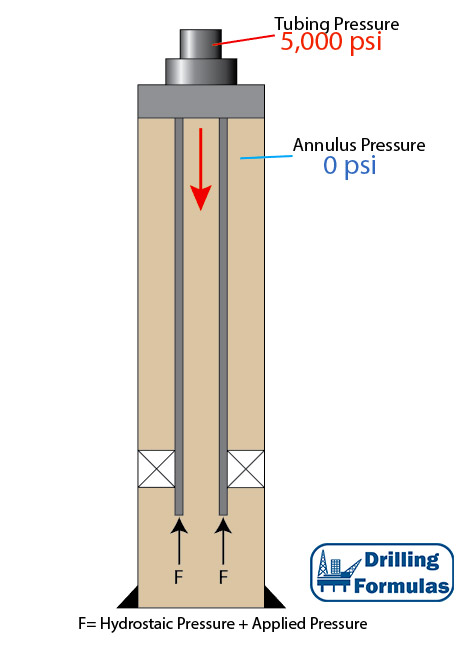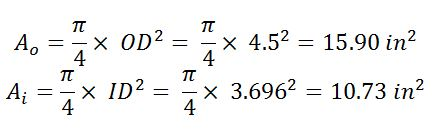# Piston Force on Open-Ended Tubular

Piston force is a load caused directly by changes in pressure acting on the exposed cross sectional area of pipe. This results in changing in length of tubular and force acting against tubular. In this article, it generally demonstrates force distribution based on a simple tubular diagram.Tubing Detail

• 5” Tubing
• ID of tubing = 3.696”
• Weight per length = 17.7 lb/ft
• Total Length = 10,000 ft
• Fluid density = 10 ppg
• Tubing is free to move in the packer
• Applied surface pressure = 5,000 psi

Figure 1 shows the wellbore schematic. Applied pressure (5,000 psi) will cause the piston effect to push the tubing. Therefore, at the bottom of tubing buoyancy and piston force will act in upwards direction (compression).Figure 1 – Wellbore Schematic

Pressure at the bottom

Pressure at the bottom (psi) = Hydrostatic Pressure (psi) + Applied Surface Pressure (psi)

Pressure at the bottom (psi) = (0.052×10×10,000) + 5,000 = 10,200 psi

Force at the bottom

Force at the bottom (lb) = Pressure at the bottom (psi) × cross sectional area of pipe (in2)Force at the bottom (lb) = 10,200 psi × (15.9 – 10.73) = 52,273 lb (compression ⇑(+))

Force at surface is a summation of tensile force caused by tubing weight and compression force acting on the cross sectional area of pipe.

Force at surface (lb) = (- 17.7 × 10,000) + (52,273) = -124,266 lb (tensile force ⇓ (-))

Figure 2 illustrates force distribution based on the given information.Figure 2 – Force Distribution Diagram

Note: for this calculation, the assigned signs and directions of force and pressure are as follows;

Compression force = ⇑(+)

Tensile force = ⇓(-)

Shorten in Length = (-)

Elongate in length = (+)

The signs are based on the Lubinski’s study.

References

Jonathan Bellarby, 2009. Well Completion Design, Volume 56 (Developments in Petroleum Science). 1 Edition. Elsevier Science.

Wan Renpu, 2011. Advanced Well Completion Engineering, Third Edition. 3 Edition. Gulf Professional Publishing.

Ted G. Byrom, 2014. Casing and Liners for Drilling and Completion, Second Edition: Design and Application (Gulf Drilling Guides). 2 Edition. Gulf Professional Publishing.

Lubinski, A., & Althouse, W. S. (1962, June 1). Helical Buckling of Tubing Sealed in Packers. Society of Petroleum Engineers. doi:10.2118/178-PA

Share the joy
Tagged , , , , , , , . Bookmark the permalink.